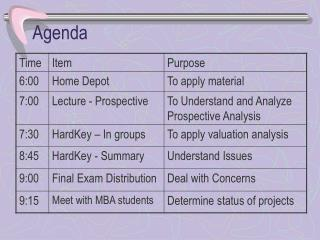DownloadDownload PresentationAgenda

Agenda

Télécharger la présentationAgenda

- - - - - - - - - - - - - - - - - - - - - - - - - - - E N D - - - - - - - - - - - - - - - - - - - - - - - - - - -
Presentation Transcript

1. Agenda

2. Home Depot • Competitive Strategy

3. Home Depot – Performance • ROE • Dupont formula • Common Size input • Asset Turnover – Inventory Turnover – Store Info • Comparison to Hechinger

4. Home Depot – Cash Flow Analysis • Negative • Invest in PPE - \$90 mil • Borrowed \$120 mil in 84, and \$92 in 85 • Heichinger

5. Cash Required for Expansion – Can we get it?????

6. Projected Income Statement

7. Projected Cash Flow Statement

8. What do we need • \$8.4 mil per store (6.6 for PPE & 1.8 mil for inv) • 9 stores * \$8.4 = \$75.6 mil • Plus \$1.4 mil • Thus need \$77 mil • What to do?

9. Financing Options • Difficult to get – maximum interest allowed is \$4.8mil at 8% - can only borrow \$60 mil • Could sale & lease back • Issue equity • Improve cash flows from operations? how

10. What did they do? • Sales grew to \$1.001 bil in 86 & 1.45 in 87 • Earnings grew to 23.8 mil and 54.1 mil 86/87 • ROS up to 2.4% and 3.7% thru better inventory control, better cost control and 38% increase in in transactions per store • Raised \$50mil through equity

11. Class 5 – Prospective Analysis • We are we going • We want to know the value of the firm • How do we define the value of any asset • Present value of future earning potential

12. Accounting Based MethodsDiscounted Cash Flows • Compute free cash flow for appropriate number of years • Compute Terminal Value (next Class) • Discount Both based upon the WACC • Add Excess Cash • Subtract debt • Gives you present Value to Equity holders

13. Accounting Based Methods Abnormal Earnings • Compute Forecasted Earning (after tax & interest) • Compute Normal earnings • Difference = Abnormal Earnings (similar to EVA) • Discount abnormal Earnings & Terminal Value • Add the Book value at the valuation date

14. Accounting Based Methods Abnormal ROE • Forecast ROE (remember it’s a RATIO) • Subtract ROE based upon normal return • Determine the cumulative growth in beg. Book value and apply to Abnormal ROE • Determine the Present value of abnormal ROE • Determine the Cumulative PV of ROE • Calculate the Terminal Value • Apply Cum. PV of Abnormal ROE to the book value of equity at the beginning